Electricity Electric Charge and Force O Electric charge

• Slides: 27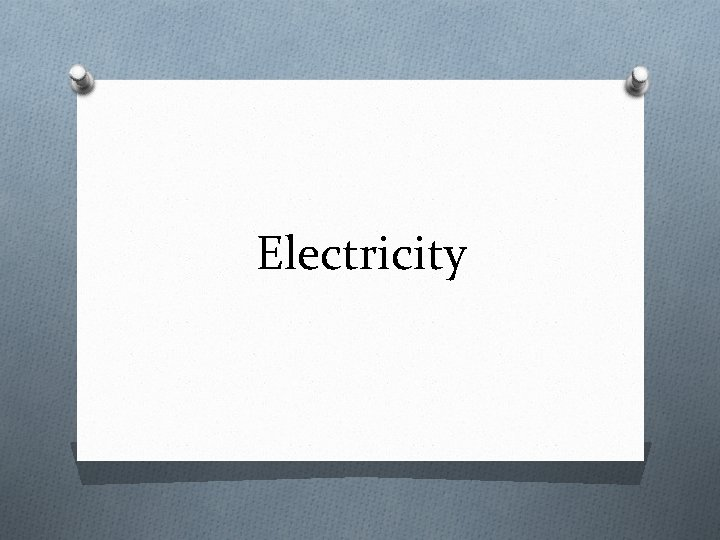ElectricityElectric Charge and Force O Electric charge: an electrical property of matter that creates electric and magnetic forces and interactions. O An object can have a negative charge, positive charge, or no charge. O Like energy, electrical charges can never be created nor destroyed. O Conservation of charge is one of the fundamental laws of nature.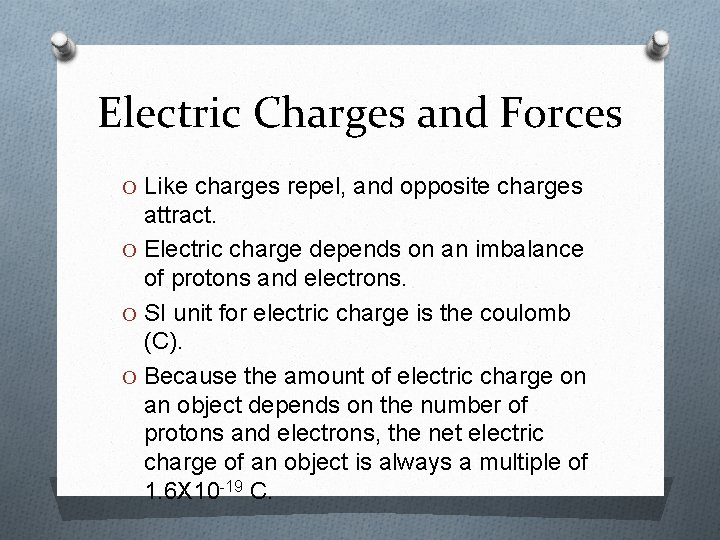Electric Charges and Forces O Like charges repel, and opposite charges attract. O Electric charge depends on an imbalance of protons and electrons. O SI unit for electric charge is the coulomb (C). O Because the amount of electric charge on an object depends on the number of protons and electrons, the net electric charge of an object is always a multiple of 1. 6 X 10 -19 C.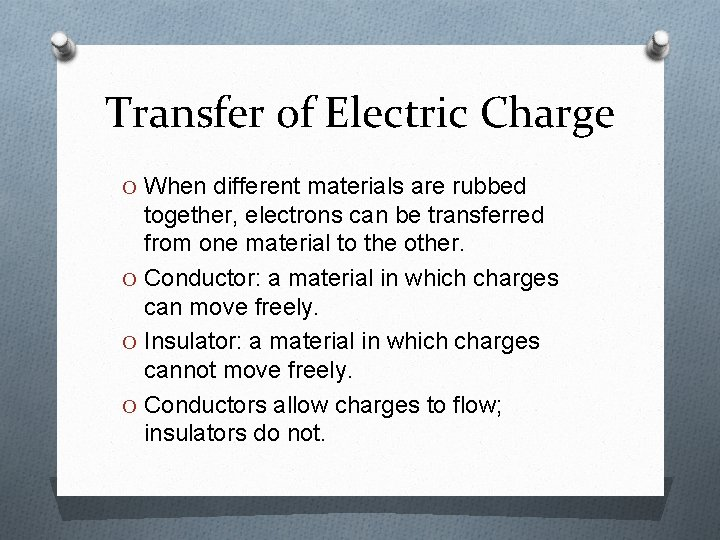Transfer of Electric Charge O When different materials are rubbed together, electrons can be transferred from one material to the other. O Conductor: a material in which charges can move freely. O Insulator: a material in which charges cannot move freely. O Conductors allow charges to flow; insulators do not.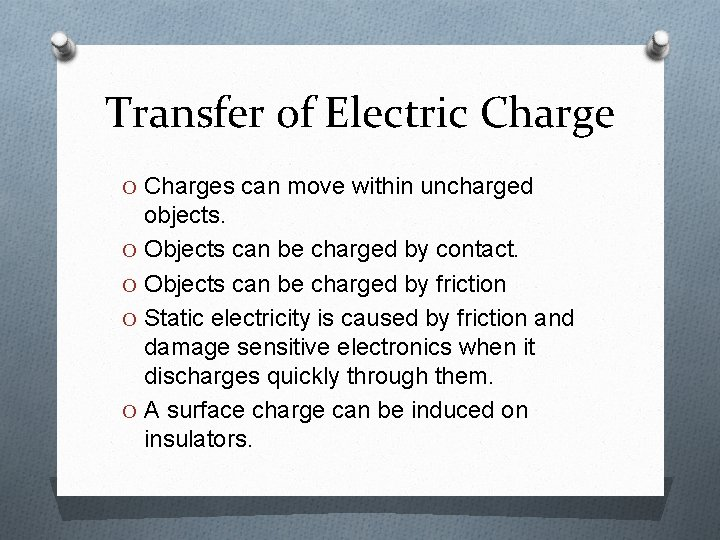Transfer of Electric Charge O Charges can move within uncharged objects. O Objects can be charged by contact. O Objects can be charged by friction O Static electricity is caused by friction and damage sensitive electronics when it discharges quickly through them. O A surface charge can be induced on insulators.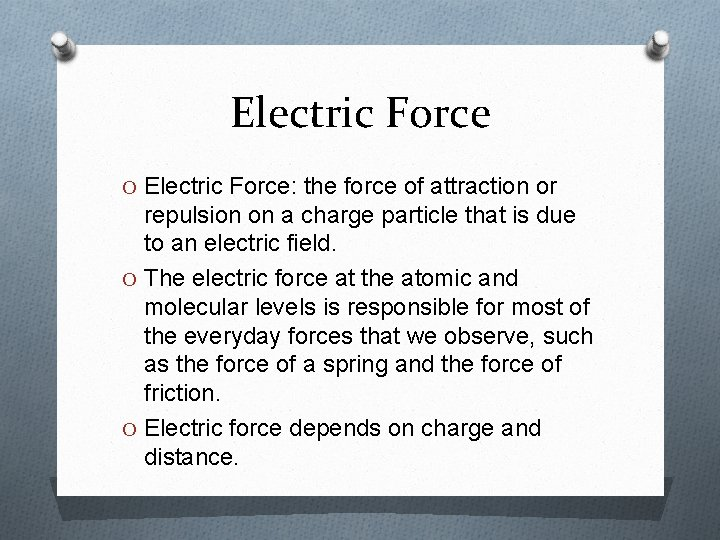Electric Force O Electric Force: the force of attraction or repulsion on a charge particle that is due to an electric field. O The electric force at the atomic and molecular levels is responsible for most of the everyday forces that we observe, such as the force of a spring and the force of friction. O Electric force depends on charge and distance.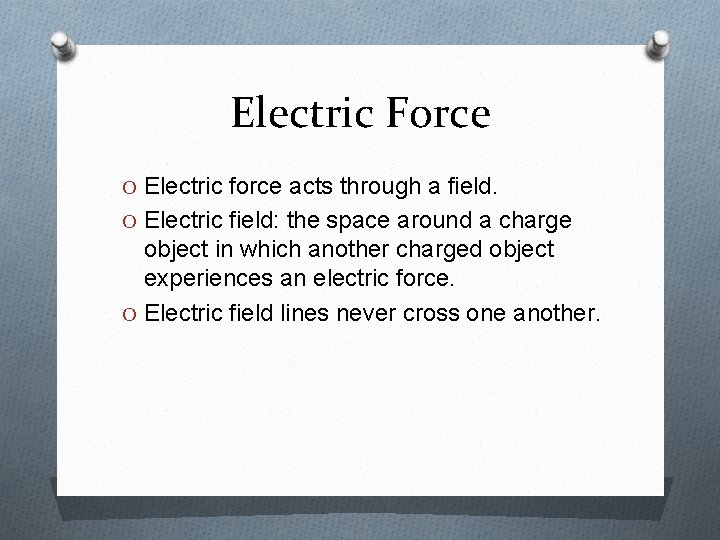Electric Force O Electric force acts through a field. O Electric field: the space around a charge object in which another charged object experiences an electric force. O Electric field lines never cross one another.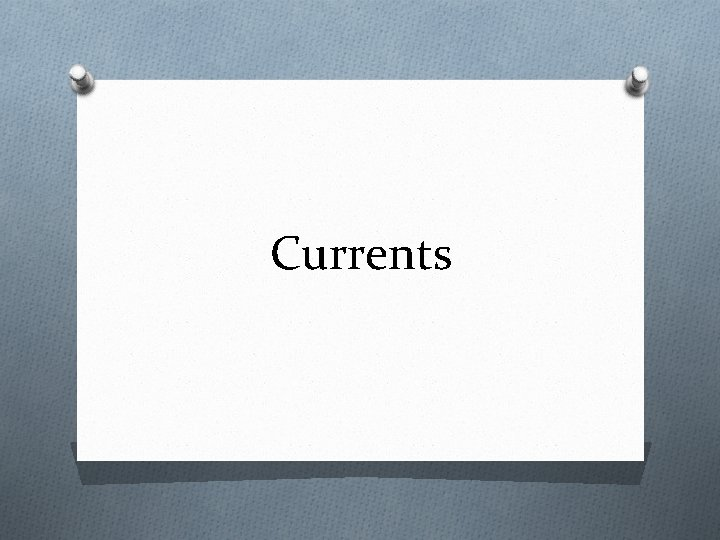Currents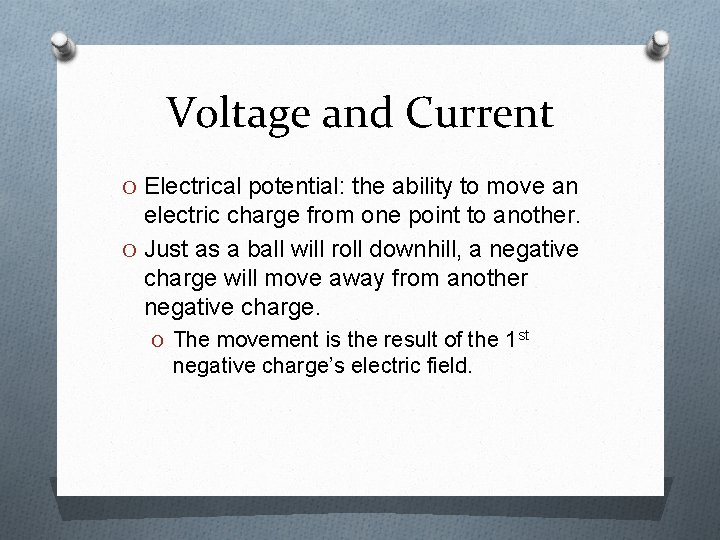Voltage and Current O Electrical potential: the ability to move an electric charge from one point to another. O Just as a ball will roll downhill, a negative charge will move away from another negative charge. O The movement is the result of the 1 st negative charge’s electric field.Voltage and Current O Potential difference is measured in volts. O Potential difference: the voltage difference in potential between two points in a circuit. (the change in the electrical potential energy of a charged particle divided by its charge. O SI unit for potential difference is volt (V) O 1 V = 1 J/C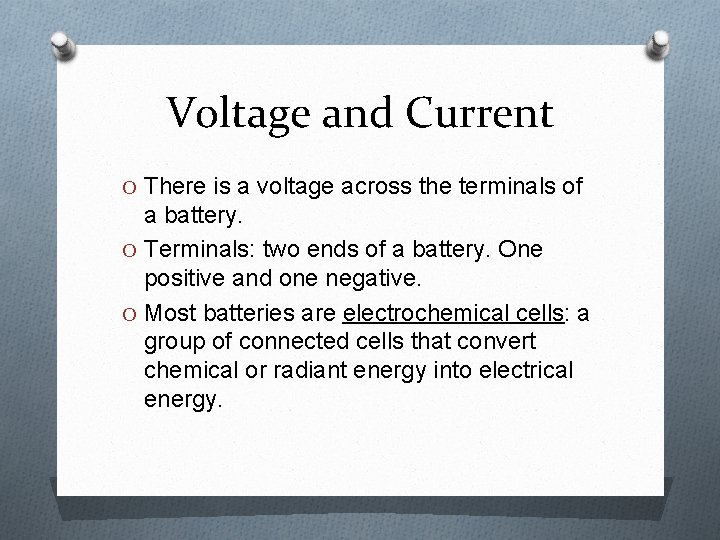Voltage and Current O There is a voltage across the terminals of a battery. O Terminals: two ends of a battery. One positive and one negative. O Most batteries are electrochemical cells: a group of connected cells that convert chemical or radiant energy into electrical energy.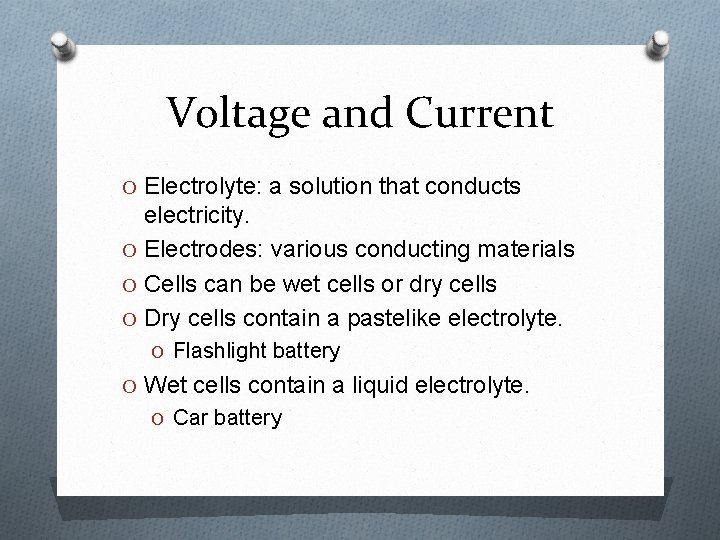Voltage and Current O Electrolyte: a solution that conducts electricity. O Electrodes: various conducting materials O Cells can be wet cells or dry cells O Dry cells contain a pastelike electrolyte. O Flashlight battery O Wet cells contain a liquid electrolyte. O Car batteryVoltage and Current O Current is the rate of charge movement. O SI unit is ampere (A). O 1 A = 1 C moving past a point in 1 s. O Direct current: charges always move from one terminal to the other in the same direction. O Conventional current: positive charge that would have the same effect as the actual motion of charge in the material.Electrical Resistance O Resistance: the opposition presented to the current by a material or device. O Resistance is caused by internal friction, which slows the movement of charges through a conducting material. O Resistance can be calculated if current and voltage are known.Electrical Resistance O Resistance = voltage/current or R=V/I O This equation is also called Ohm’s Law. O SI unit for resistance is ohm (Ω) O 1 Ω = 1 V / A O Resistor: a special type of conductor used to control current. O Every resistor is designed to have a specific resistance.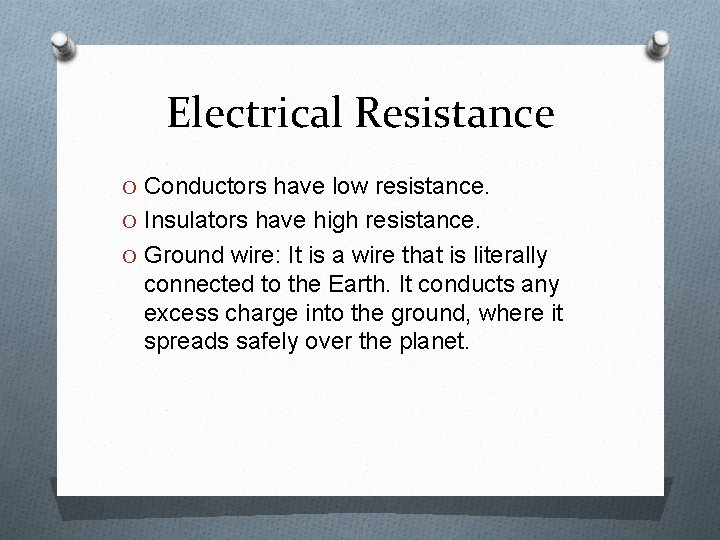Electrical Resistance O Conductors have low resistance. O Insulators have high resistance. O Ground wire: It is a wire that is literally connected to the Earth. It conducts any excess charge into the ground, where it spreads safely over the planet.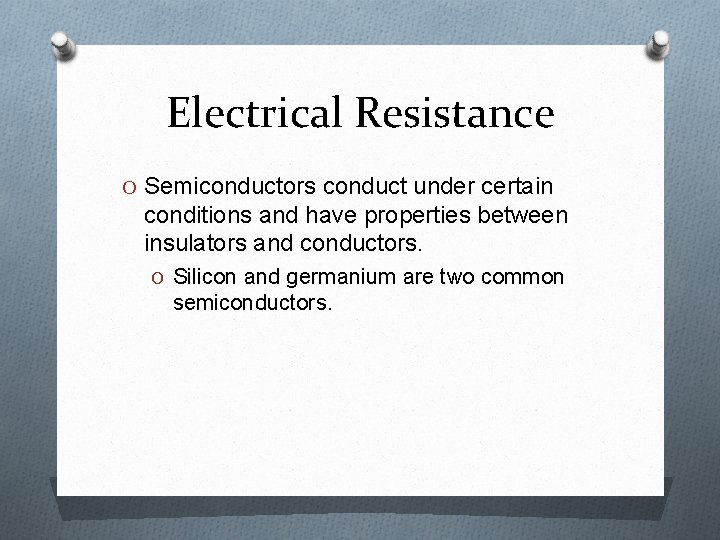Electrical Resistance O Semiconductors conduct under certain conditions and have properties between insulators and conductors. O Silicon and germanium are two common semiconductors.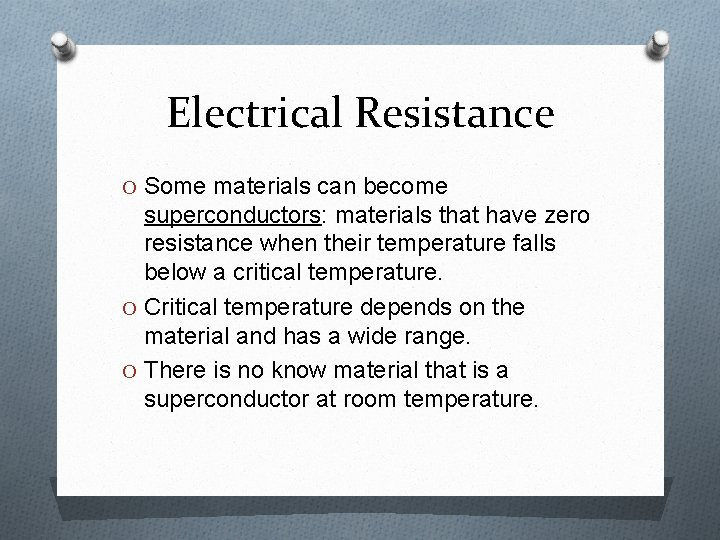Electrical Resistance O Some materials can become superconductors: materials that have zero resistance when their temperature falls below a critical temperature. O Critical temperature depends on the material and has a wide range. O There is no know material that is a superconductor at room temperature.Circuits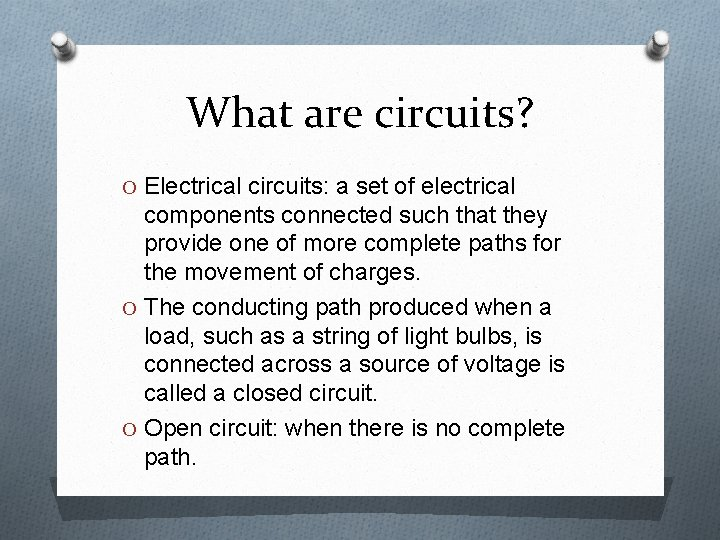What are circuits? O Electrical circuits: a set of electrical components connected such that they provide one of more complete paths for the movement of charges. O The conducting path produced when a load, such as a string of light bulbs, is connected across a source of voltage is called a closed circuit. O Open circuit: when there is no complete path.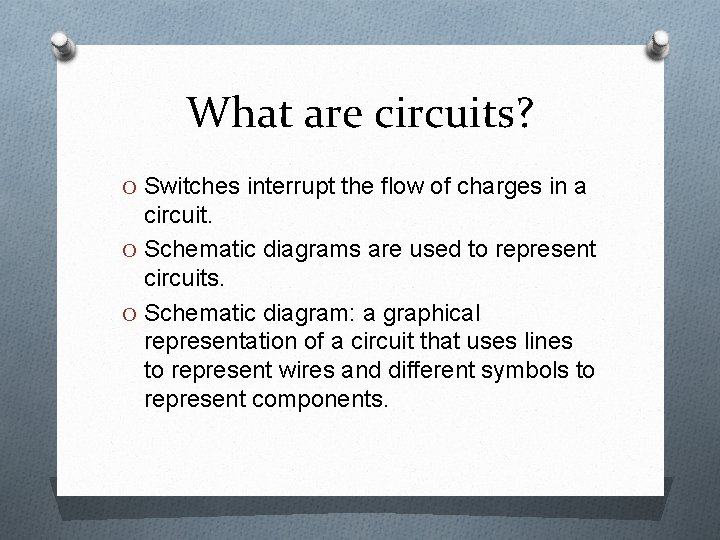What are circuits? O Switches interrupt the flow of charges in a circuit. O Schematic diagrams are used to represent circuits. O Schematic diagram: a graphical representation of a circuit that uses lines to represent wires and different symbols to represent components.Series and Parallel Circuits O Electrical devices can be connected as a series circuit so that the voltage is divided among the devices. They can also be connected as a parallel circuit so that voltage is the same across each device. O Series circuits have a single path for current. O Parallel circuits have multiple paths for current.Electrical Energy & Electrical Power O Some of this energy is transformed into useful work, such as the turning of a motor, and some is lost as heat. O Electric power: the rate at which electrical energy is converted into other forms of energy. O Power = current X voltage or P = IV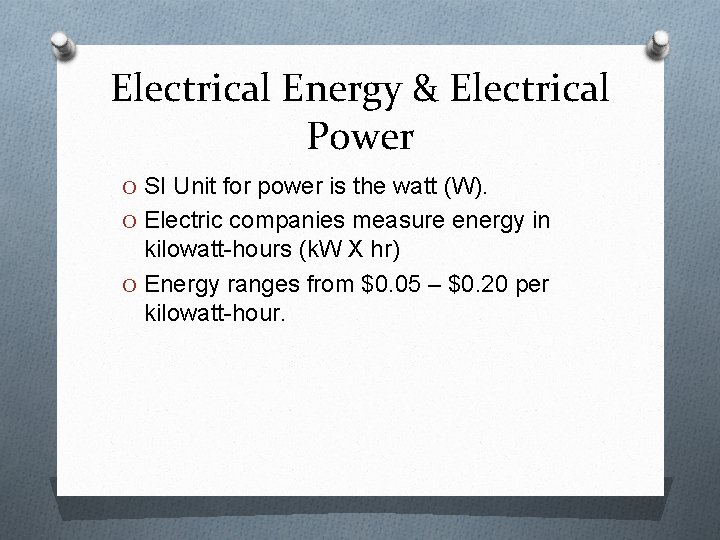Electrical Energy & Electrical Power O SI Unit for power is the watt (W). O Electric companies measure energy in kilowatt-hours (k. W X hr) O Energy ranges from \$0. 05 – \$0. 20 per kilowatt-hour.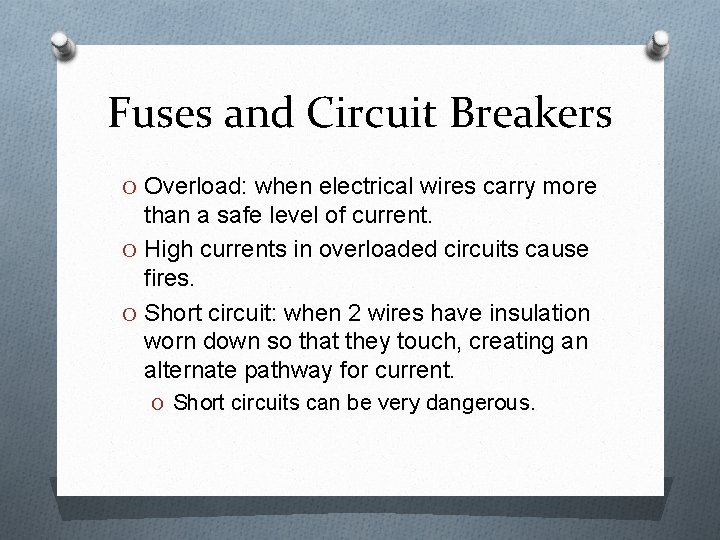Fuses and Circuit Breakers O Overload: when electrical wires carry more than a safe level of current. O High currents in overloaded circuits cause fires. O Short circuit: when 2 wires have insulation worn down so that they touch, creating an alternate pathway for current. O Short circuits can be very dangerous.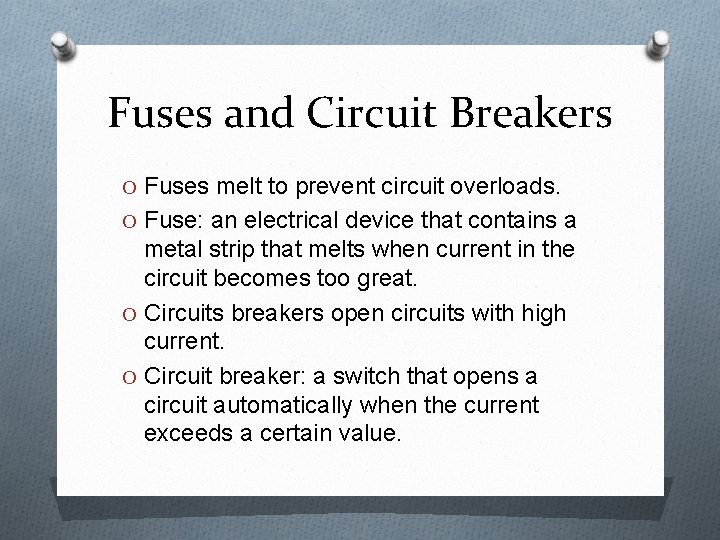Fuses and Circuit Breakers O Fuses melt to prevent circuit overloads. O Fuse: an electrical device that contains a metal strip that melts when current in the circuit becomes too great. O Circuits breakers open circuits with high current. O Circuit breaker: a switch that opens a circuit automatically when the current exceeds a certain value.Fuses and Circuit Breakers O Bimetallic strip: a strip with two different metals welded together that responds to circuit overload by opening the circuit. O Ground Fault Circuit Interrupter (GFCI): a special kind of electrical outlet that acts as a small circuit breaker , usually found near water sources like bathrooms or kitchens.